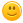#I need to use the number pi in C. How to enter it?

closed Nov 25
for example y=pi*4?

+1 vote
answered Nov 19 by (40,320 points)

Well, you know the value of PI, all you have to do is create either a variable or a macro for it.

```#include <stdio.h>

#define PI 3.14159265359f
// float PI = 3.14159265359f; // This would work just as fine

int main()
{
float y = PI * 4.0f;
printf("y = %f\n", y);
return 0;
}```
commented Nov 19 by (100 points)
i agree with you since you declared the value of PI as a global var it can be used anywhere in the prorgram
answered Nov 24 by (140 points)

You can also use it directly by adding another library in your code.

The library is math.h, I will demonstrate it by calculating area of a circle, so it would look like,

#include<stdio.h>

#include<math.h>

int main()

{

float r;

printf("Enter the value of radius of circle\n");

scanf("%f",&r);

printf("The area of the circle is %f\n",M_PI*r*r);

return 0;

}

Hope it helps